## Links for this tutorial

Data, examples and exercises

Slides

## Scope of this tutorial

The emphasis of this tutorial will be on:

• Open source tools for using geospatial data in python
• "Pythonic" tools for working with data
• Interfacing with NumPy and the SciPy stack
• Hands-on examples and exercises

## Outside the scope of this tutorial

Some big topics we will not be covering (much):

• Geospatial analysis
• Plotting on maps
• Web mapping tools
• PostGIS (or other geospatial databases)
• Full GIS applications (e.g. ArcGIS and QGIS)

## Outline

• Reading vector data with Fiona
• Geometry with Shapely
• Brief interlude on map projections
• Rasterio
• GeoPandas

## Vector and raster data

Vector data includes points, lines, polygons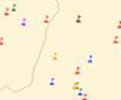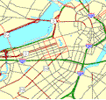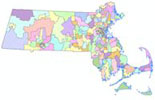Raster data includes images, digital elevation models, 2-D fields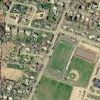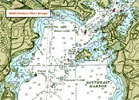## GeoJSON

```{
"type": "Feature",
"geometry": {
"type": "Point",
"coordinates": [125.6, 10.1]
},
"properties": {
"name": "Dinagat Islands"
}
}
```

Represents simple features in a JSON format.

A GeoJSON object can represent any of the following:

• A `geometry` (Point, LineString, Polygon, MultiPoint, MultiLineString, MultiPolygon, or GeometryCollection)
• A feature (object with `geometry` and `properties`)
• A collection of features

## GDAL

GDAL (Geospatial Data Abstraction Library) is the open source Swiss Army knife of raster formats. It also includes the OGR simple features library for vector formats.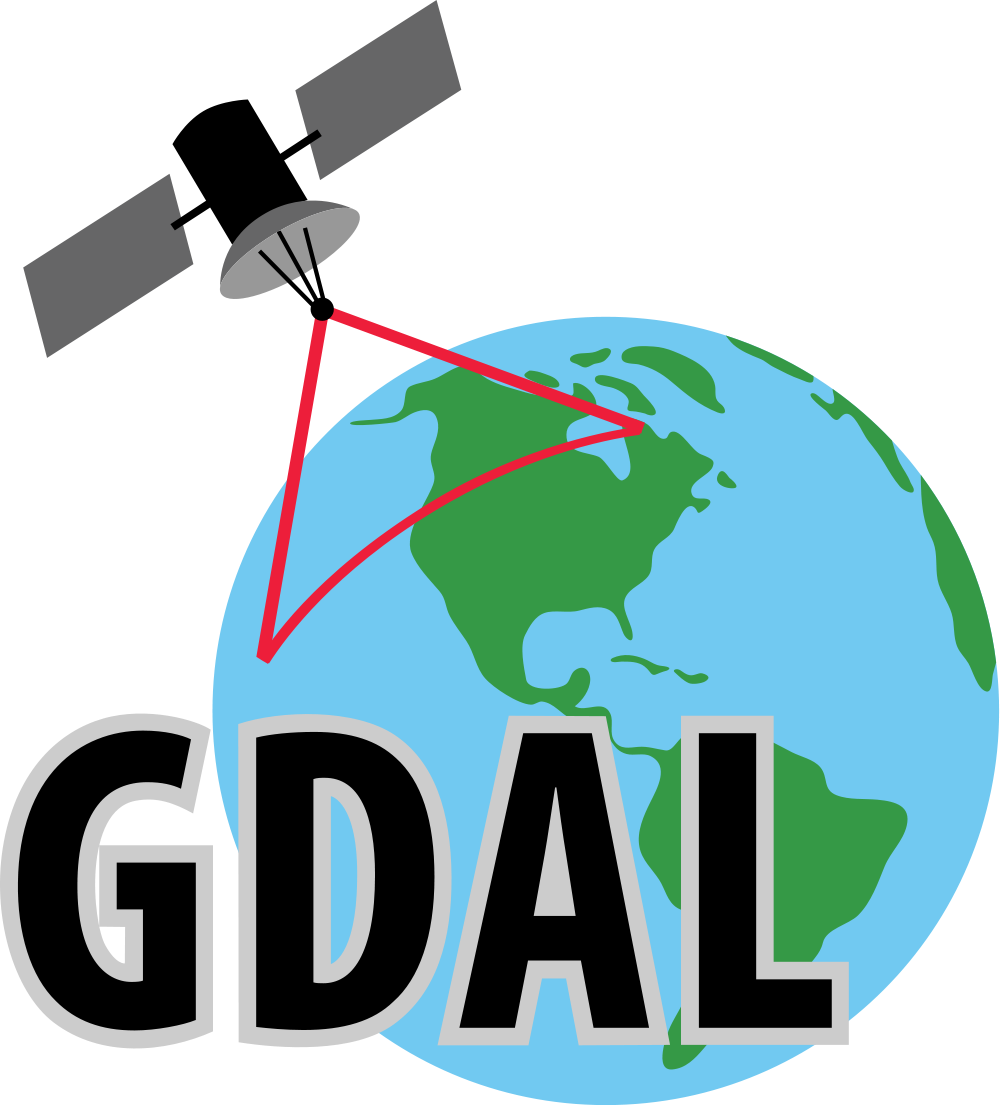GDAL's python bindings expose most of the functionality of GDAL.

```>>> from osgeo import gdal
>>> from osgeo import ogr
```

## But...

GDAL python bindings are not very "pythonic"

```geo = gdal.Open(raster_file)
drv = geo.GetDriver()
```

Most of the GDAL bindings are thin wrappers of their C++ counterparts.

See `examples/gdal_example.py`

OGR → Fiona

GDAL → Rasterio

## Fiona

Fiona is a minimalist python package for reading (and writing) vector data in python. Fiona provides python objects (e.g. a dictionary for each record) to geospatial data in various formats.

```>>> import fiona
>>> c = fiona.open('data/test_uk.shp')
>>> rec = c.next()
>>> rec.keys()
{'AREA': 244820.0,
'CAT': 232.0,
'CNTRY_NAME': u'United Kingdom',
'FIPS_CNTRY': u'UK',
'POP_CNTRY': 60270708.0}
```
```>>> rec['geometry']
{'coordinates': [[(0.899167, 51.357216),
(0.885278, 51.35833),
(0.7875, 51.369438),
(0.781111, 51.370552),
(0.904722, 51.358055),
(0.899167, 51.357216)]],
'type': 'Polygon'}
```
examples/reading vector data with Fiona.ipynb

## Fiona command line interface

```Usage: fio [OPTIONS] COMMAND [ARGS]...

Commands:
cat      Concatenate and print the features of datasets
collect  Collect a sequence of features.
dump     Dump a dataset to GeoJSON.
info     Print information about a dataset.
insp     Open a dataset and start an interpreter.
load     Load GeoJSON to a dataset in another format.
```

## Exercise: reading a shapefile with Fiona

`exercises/Fiona exercise.ipynb`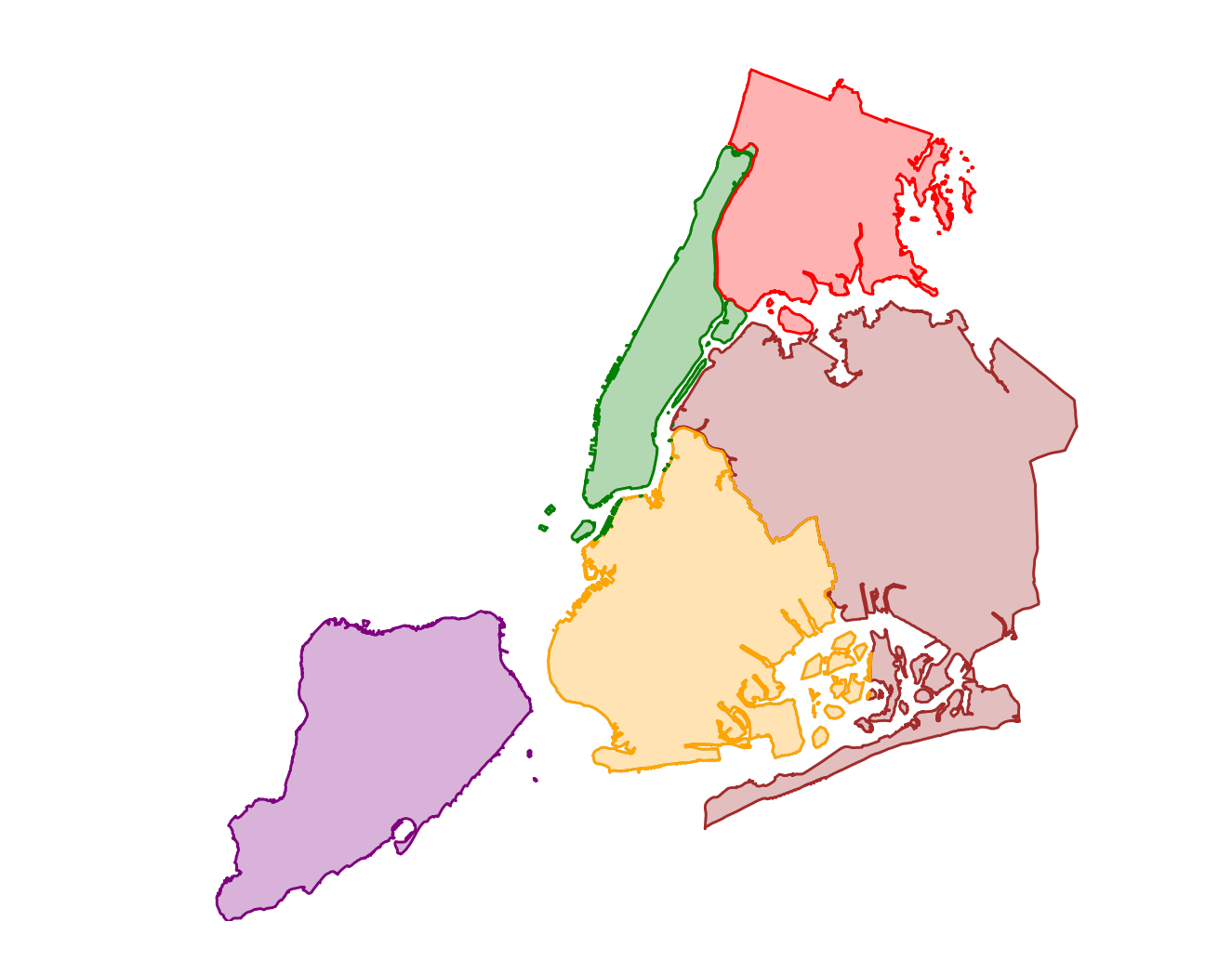## Shapely

Shapely is a python library for geometric operations using the GEOS library.

Shapely can perform:

• geometry validation
• geometry creation (e.g. collections)
• geometry operations

## Shapely geometric operations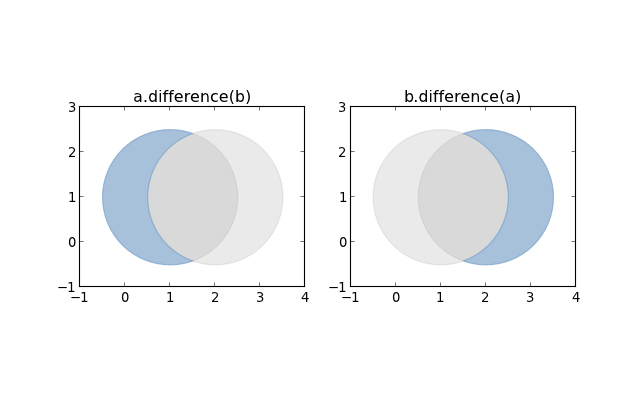## Shapely geometric operations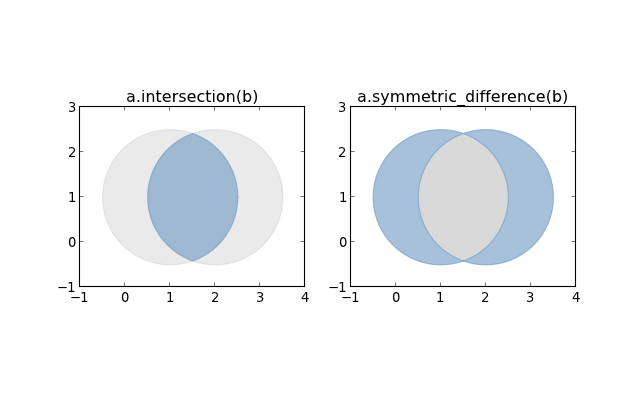## Shapely geometric operations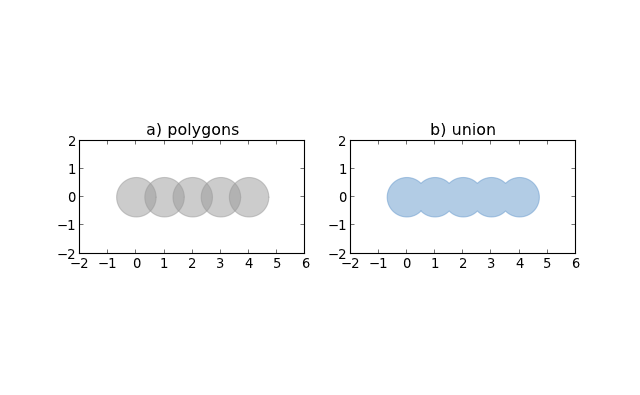## Shapely geometric operations## Dilating a line

```>>> line = LineString([(0, 0), (1, 1), (0, 2), (2, 2), (3, 1), (1, 0)])
>>> dilated = line.buffer(0.5)
>>> eroded = dilated.buffer(-0.3)
```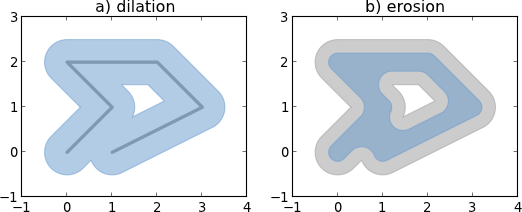Source: Shapely documentation

## Binary predicates

`object.almost_equals(other[, decimal=6])`

`object.contains(other)`

`object.crosses(other)`

`object.disjoint(other)`

`object.equals(other)`

`object.intersects(other)`

`object.touches(other)`

`object.within(other)`

## Coordinate tuples and x, y sequences

Zip is your friend! Use it with tuple unpacking to change between sequences of (x, y) pairs and seperate x and y sequences.

```>>> pts = [(0, 0), (1, 0), (1, 1), (2, 1), (2, 2)]
>>> x, y = zip(*pts)
>>> print(x, y)
(0, 1, 1, 2, 2) (0, 0, 1, 1, 2)
```

Also, instead of calling `f(x, y)`, you can just use

```>>> f(*zip(*pts))
```

And when size matters,

```>>> from itertools import izip
```

## Exercise: Shapely

`exercises/Shapely exercise.ipynb`

## Map Projections## What's wrong with this picture?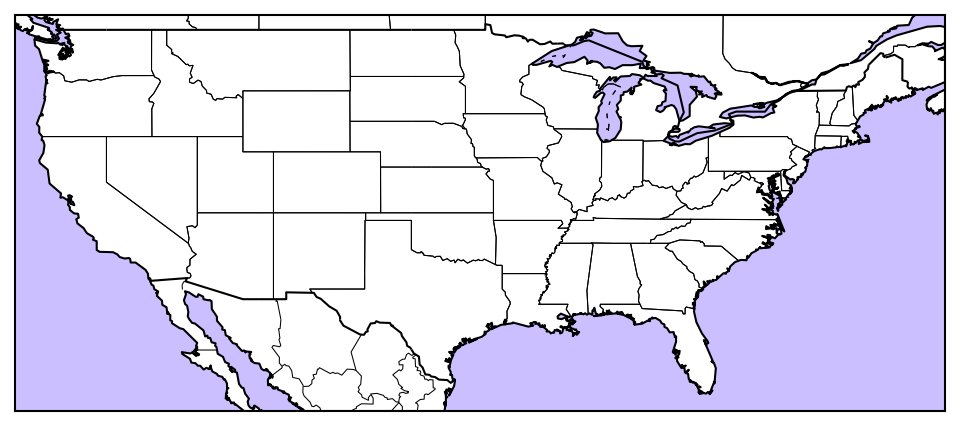## An improvement?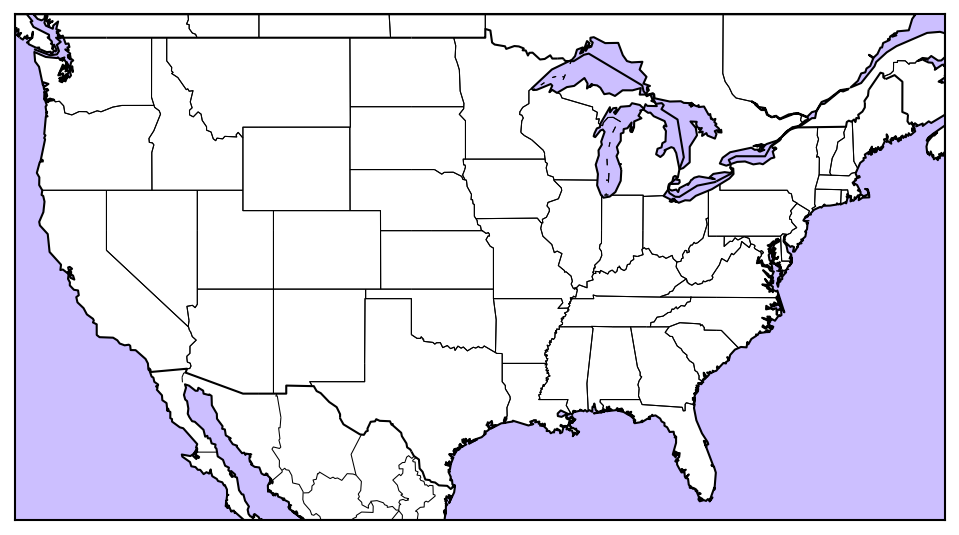## Now we're talking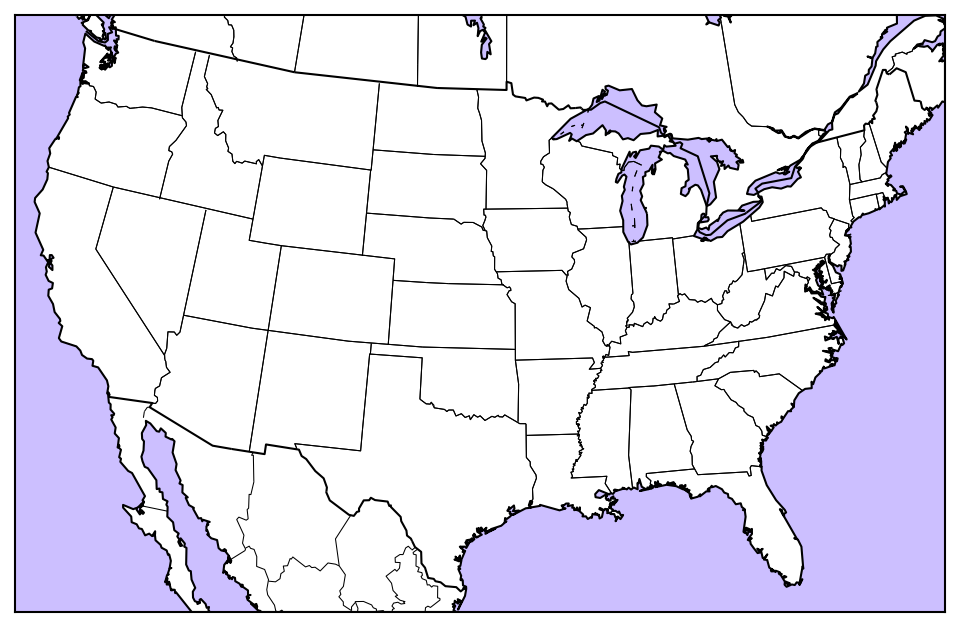## Or preserve area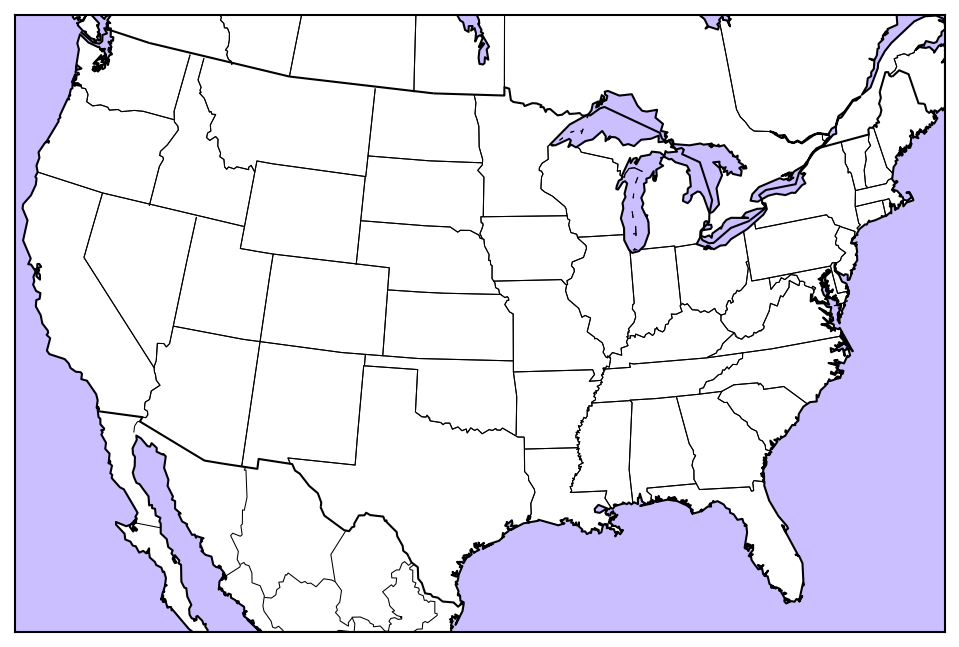## Map Projections"When people thought the earth was flat, they were wrong. When people thought the earth was spherical, they were wrong. But if you think that thinking the earth is spherical is just as wrong as thinking the earth is flat, then your view is wronger than both of them put together." -- Isaac Asimov

## Spatial Reference Systems

Examples:

• EPSG:4326 latitude, longitude in WGS-84 coordinate system
• EPSG:900913 and EPSG:3857 Google spherical Mercator
• ESRI:102718 NAD 1983 StatePlane New York Long Island FIPS 3104 Feet

Create an SRS with pyproj:

```>>> from pyproj import Proj
>>> p = Proj(init='epsg:3857')
>>> p.srs
'+units=m +init=epsg:3857 '
>>> p(-97.75, 30.25)
(-10881480.225042492, 3535725.659799159)
>>> p(-10881480.225042492, 3535725.659799159, inverse=True)
(-97.75, 30.25)
```

## pyproj example

`examples/pyproj example.ipynb`

## Rasterio python API

```with rasterio.open('manhattan.tif') as f:
imshow(img, cmap='gray')
```## rio command line tool

```\$ rio insp --ipython manhattan.tif
Rasterio 0.24.1 Interactive Inspector (Python 2.7.9)
In : src.crs
Out: {'init': u'epsg:26918'},

In : src.height, src.width
Out: (5205, 5757)

In : grd = src.read(1)

In : grd.shape
Out: (5205, 5757)
```

## Rasterio example

`examples/warp radar.ipynb`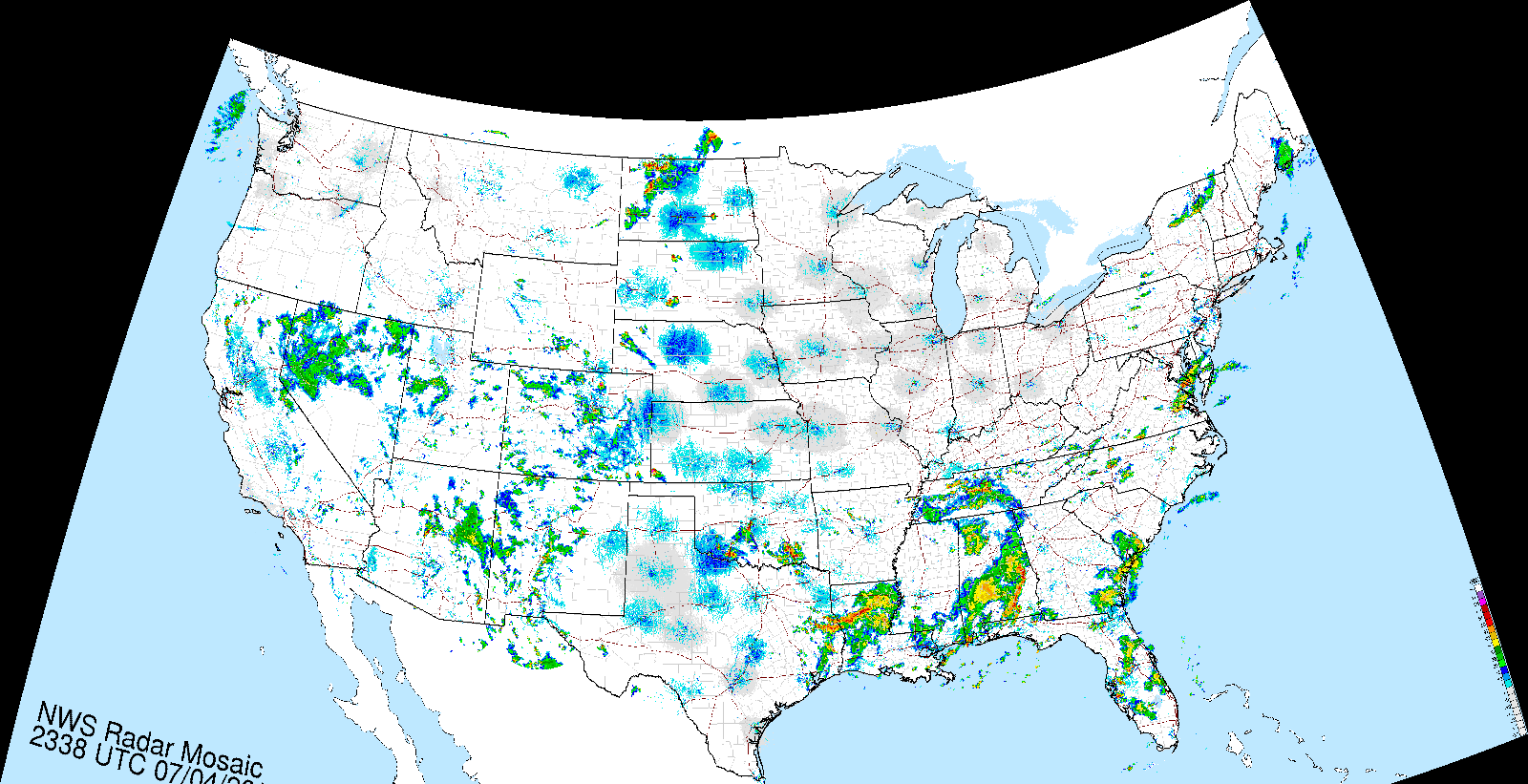## Rasterize vector features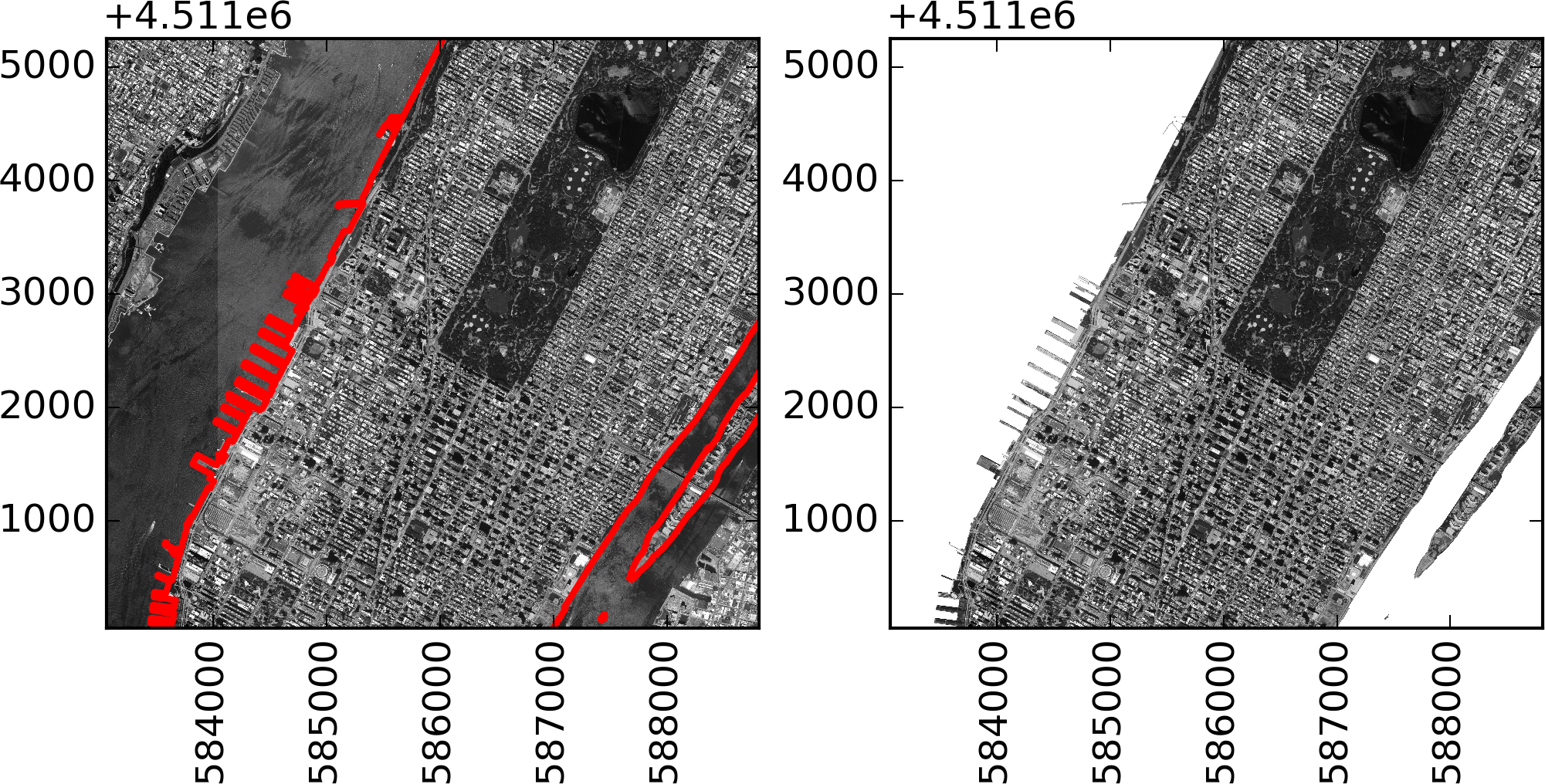`examples/rasterio_mask.py`

## Rasterizing a feature

```from rasterio.features import rasterize
mask = rasterize([poly], transform=src.transform, out_shape=src.shape)
```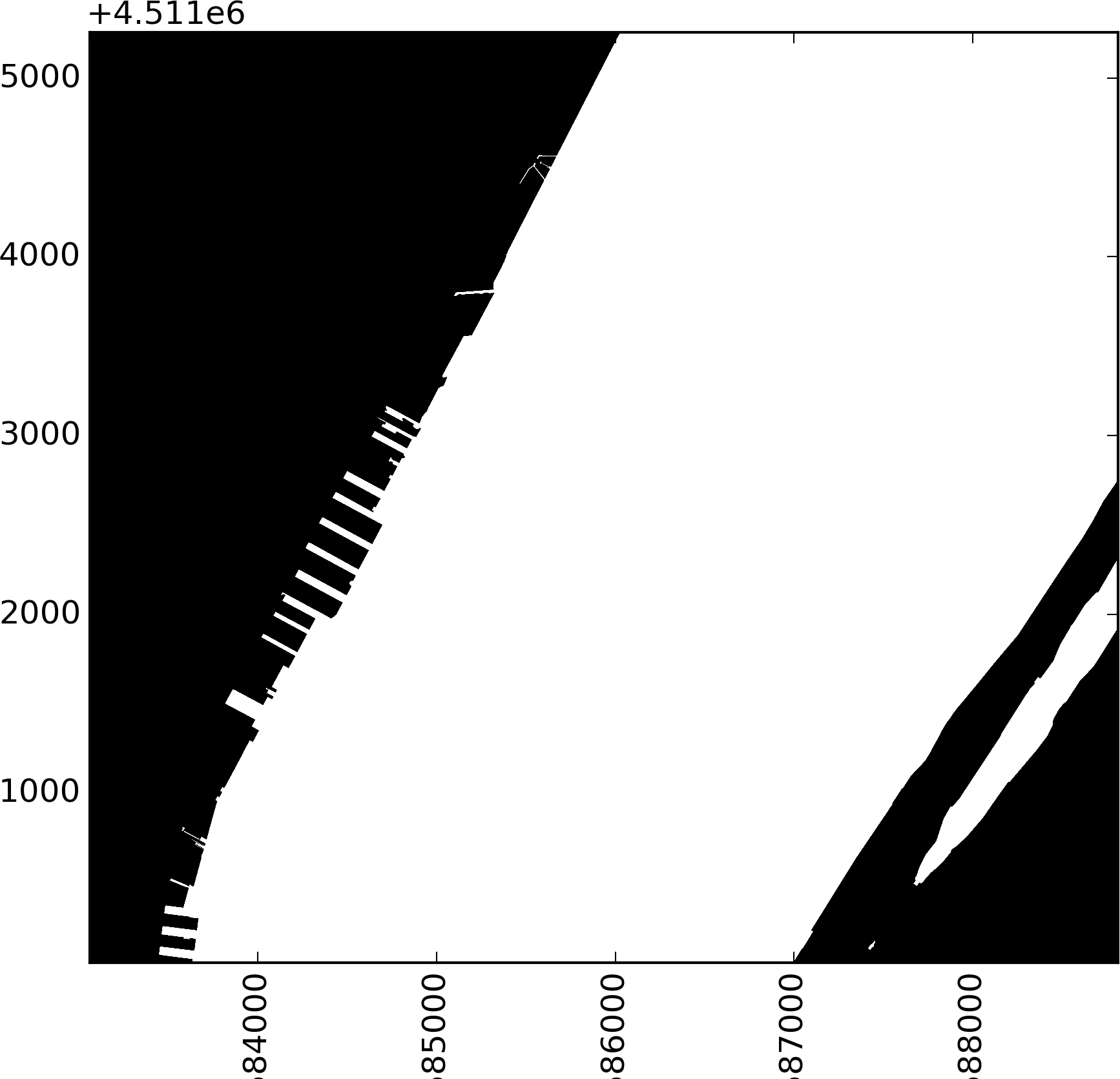`examples/rasterio_mask.py`

## Rasterio and Cartopy

```utm18n = ccrs.UTM(18)
ax = plt.axes(projection=utm18n)
extent=(left, right, bottom, top), cmap='gray')
ax.coastlines(resolution='10m', linewidth=4, color='red')
gl = ax.gridlines(linewidth=2, color='lightblue', alpha=0.5, linestyle='--')
```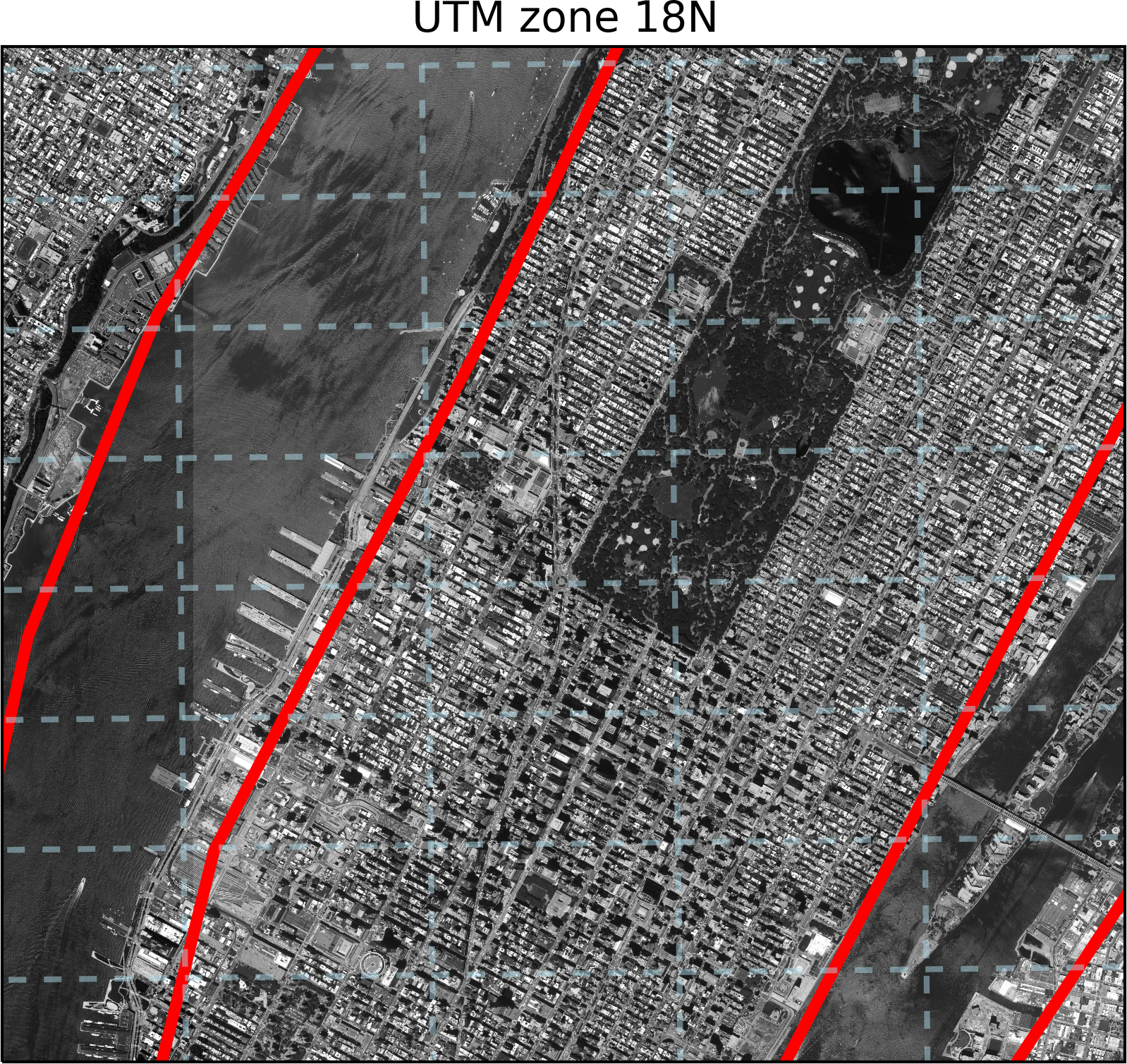`examples/rasterio_cartopy.py` (see also Cartopy docs)

## Rasterio exercise

`exercises/landsat exercise.ipynb`

## GeoPandas

• Make working with geographic data like working with other kinds of data in python
• Work with existing tools
• Desktop GIS (ArcGIS, QGIS)
• Geospatial databases (e.g., PostGIS)
• Web maps (Leaflet, D3, etc.)
• Python data tools (pandas, numpy, etc.)GeoPandas is pure python (2.6, 2.7, 3.3+)

GeoPandas depends on:

• Pandas (0.13 and up)
• Shapely (GEOS)
• Fiona (GDAL/OGR)
• Pyproj (PROJ.4)
• Matplotlib (and Descartes)
• psycopg2, sqlalchemy, geopy, rtree (optional)

## GeoPandas can do:

• Geometry operations (Shapely)
• Data alignment (pandas)
• Coordinate transformations (pyproj)
• Read/write GIS file formats (Fiona)
• Create a GeoDataFrame from PostGIS table
• Output any object as geoJSON
• Plotting

## GeoPandas Data Structures:

Pandas

• Series (1-D)
• DataFrame (2-D table)
• Panel (3-D)

GeoPandas

• GeoSeries (1-D)
contains geometry only
• GeoDataFrame (2-D)
contains a geometry column
• ?

```>>> boros = GeoDataFrame.from_file('nybb.shp')
>>> boros.set_index('BoroCode', inplace=True)
>>> boros.sort()
BoroName    Shape_Area     Shape_Leng  \
BoroCode
1             Manhattan  6.364422e+08  358532.956418
2                 Bronx  1.186804e+09  464517.890553
3              Brooklyn  1.959432e+09  726568.946340
4                Queens  3.049947e+09  861038.479299
5         Staten Island  1.623853e+09  330385.036974

geometry
BoroCode
1         (POLYGON ((981219.0557861328125000 188655.3157...
2         (POLYGON ((1012821.8057861328125000 229228.264...
3         (POLYGON ((1021176.4790039062500000 151374.796...
4         (POLYGON ((1029606.0765991210937500 156073.814...
5         (POLYGON ((970217.0223999023437500 145643.3322...
```

## Plotting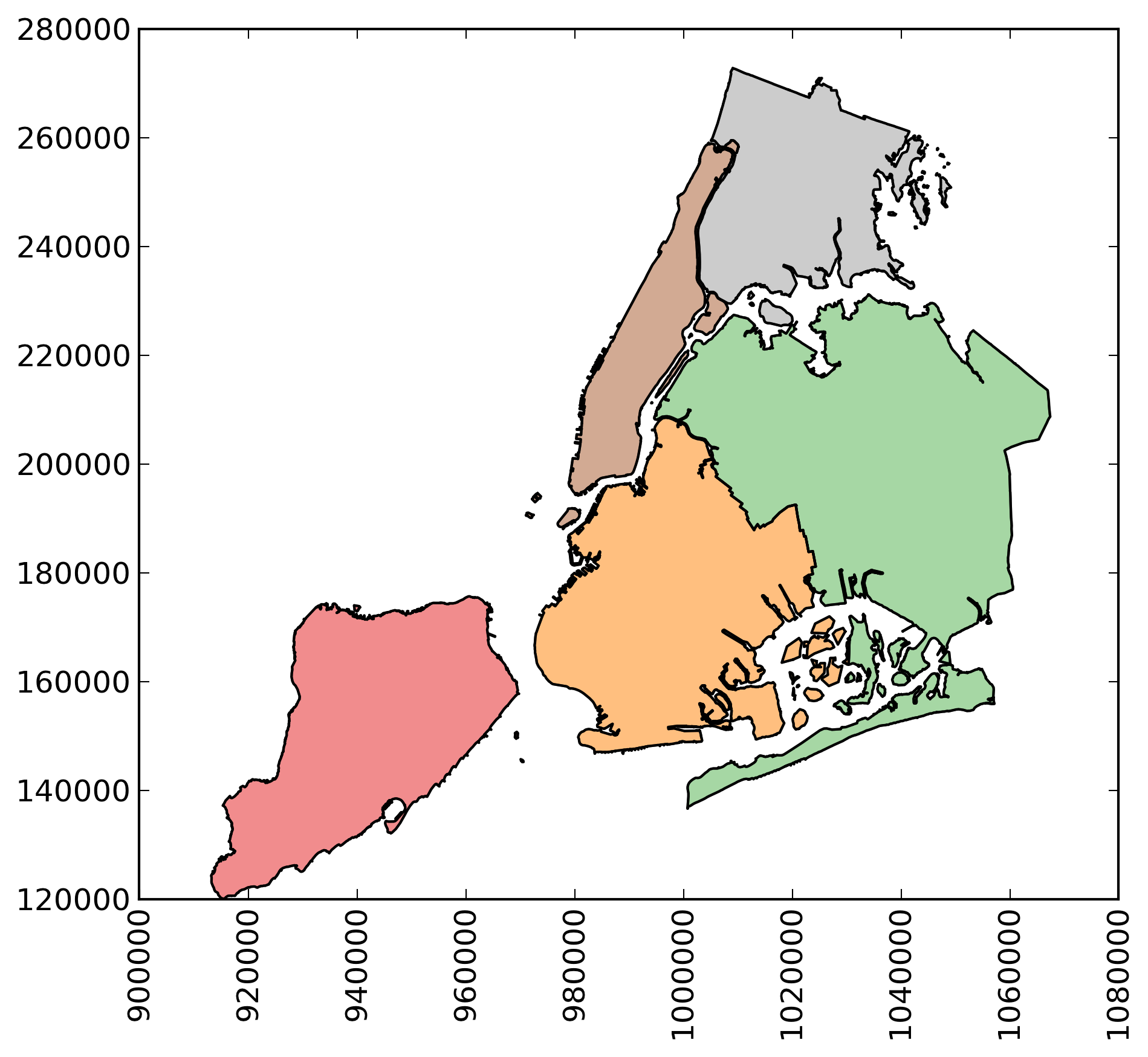boros.plot()

## Convex Hull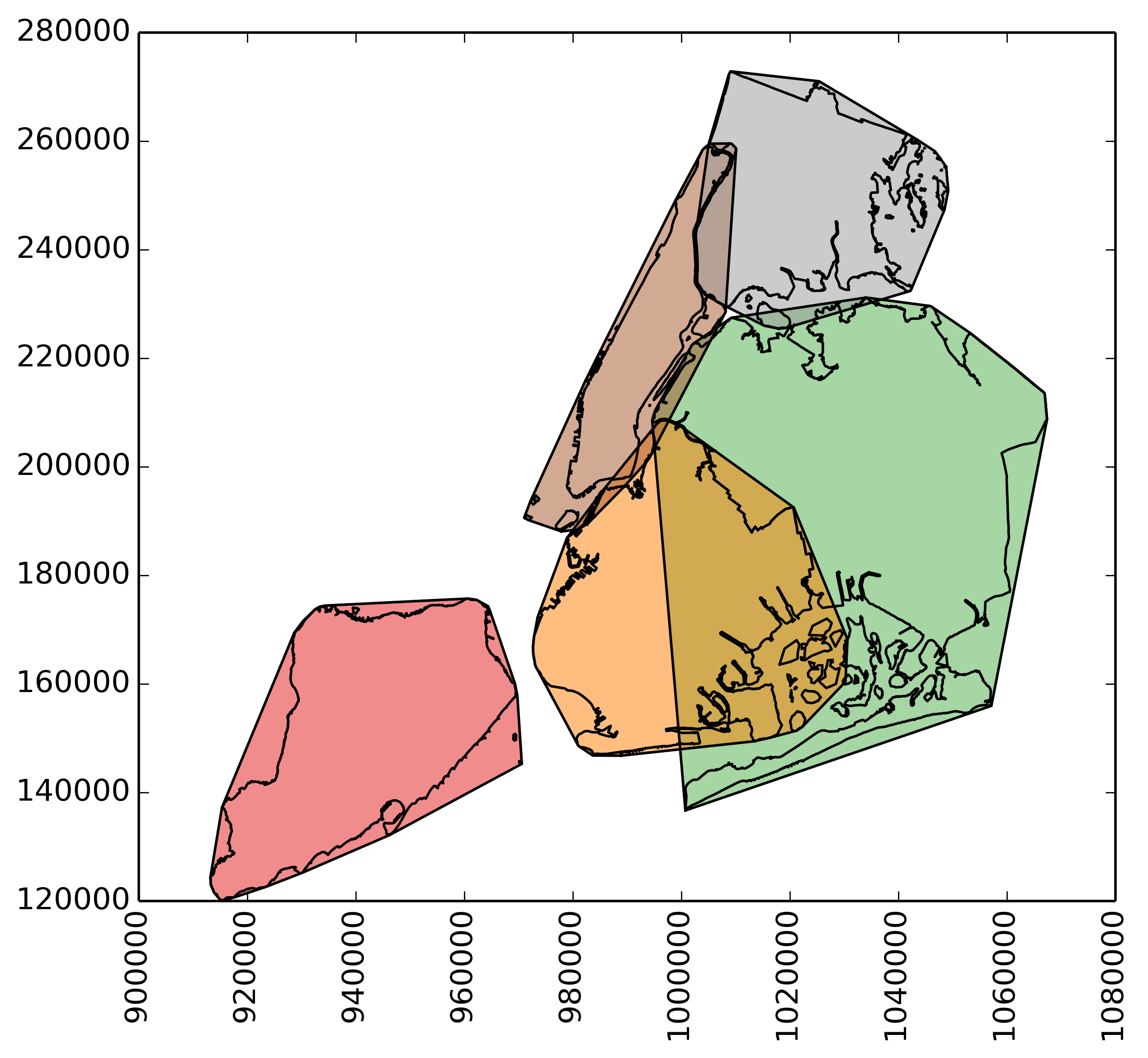boros.convex_hull.plot()

## Buffers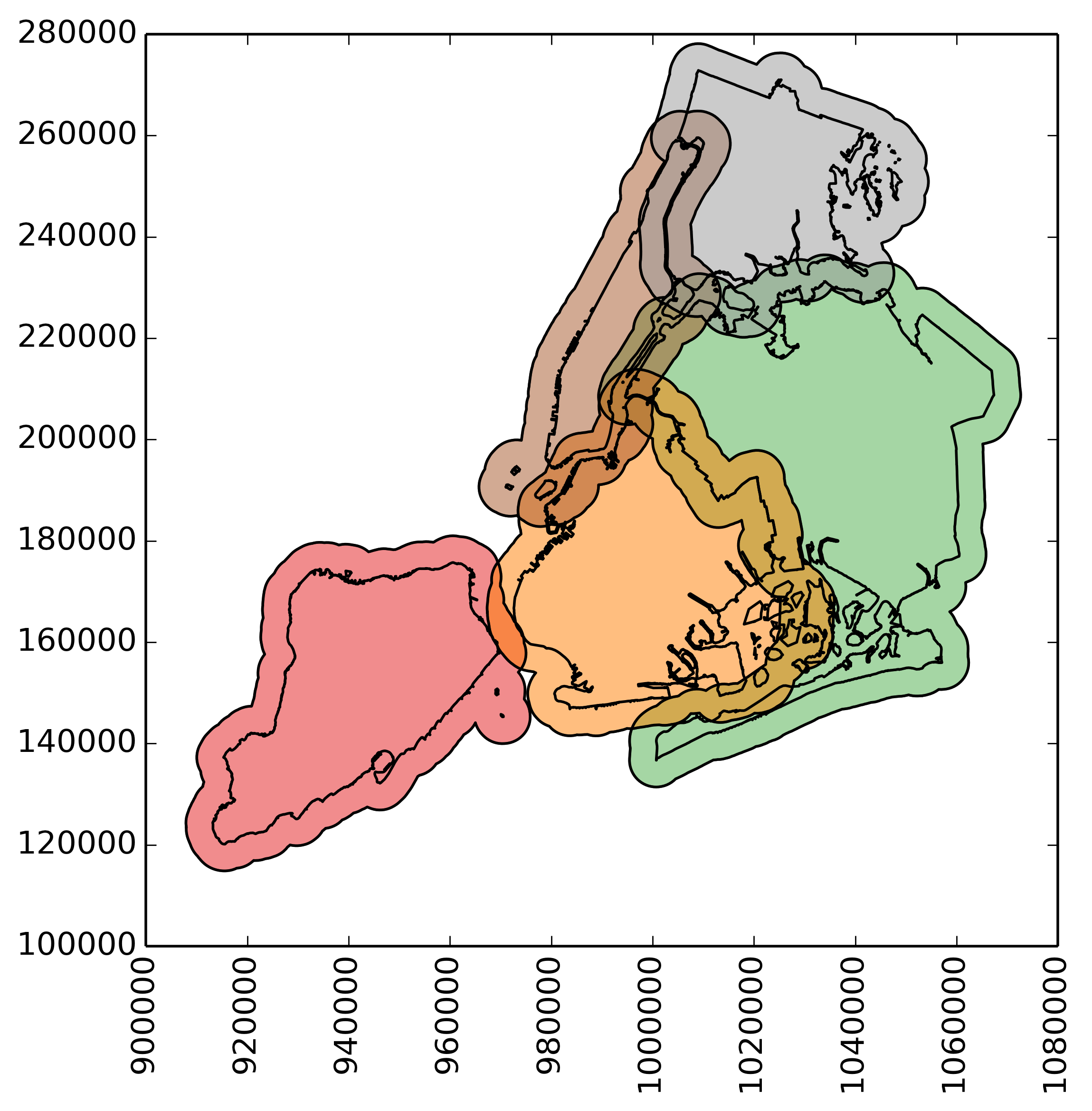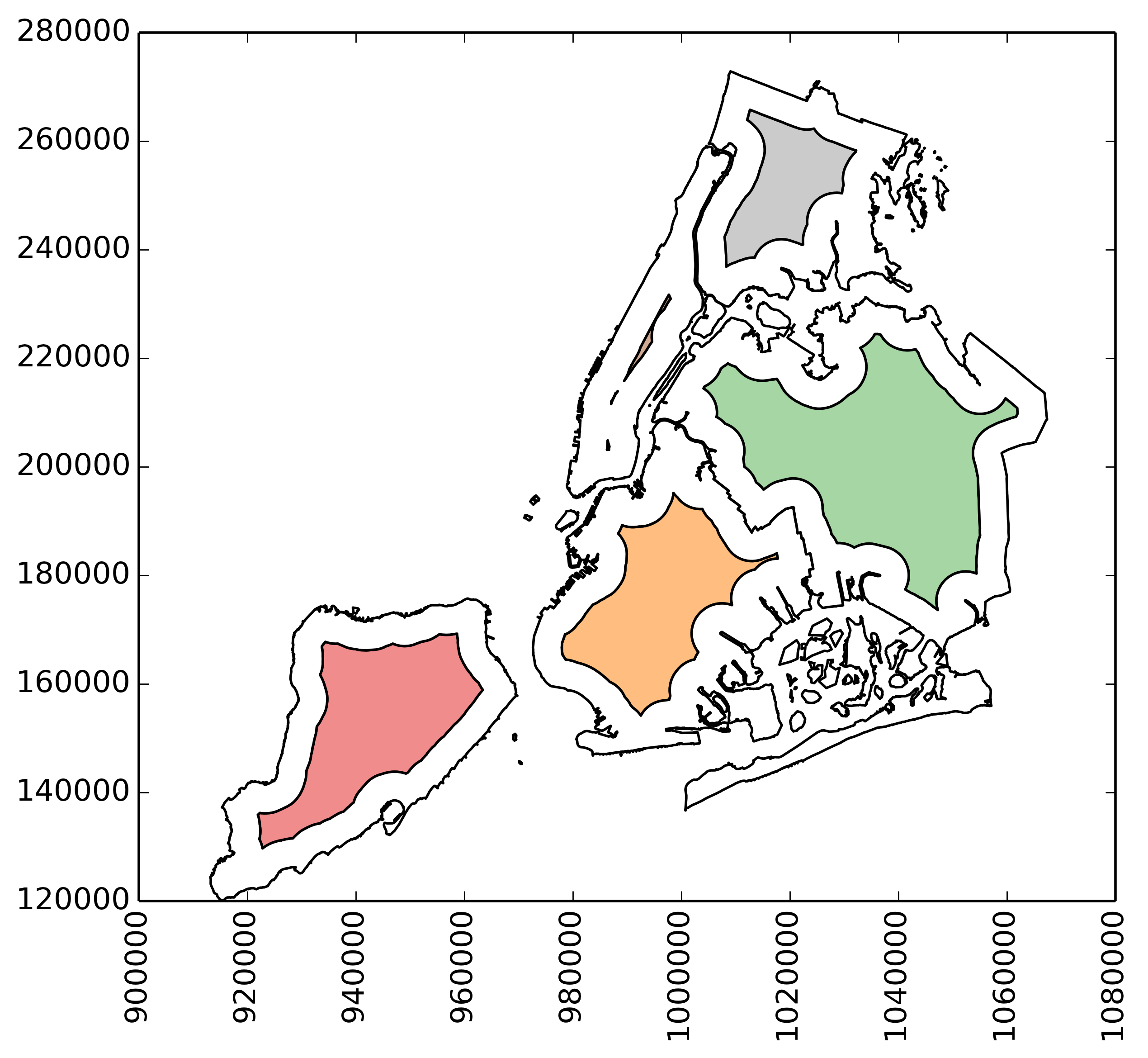## Output to GeoJSON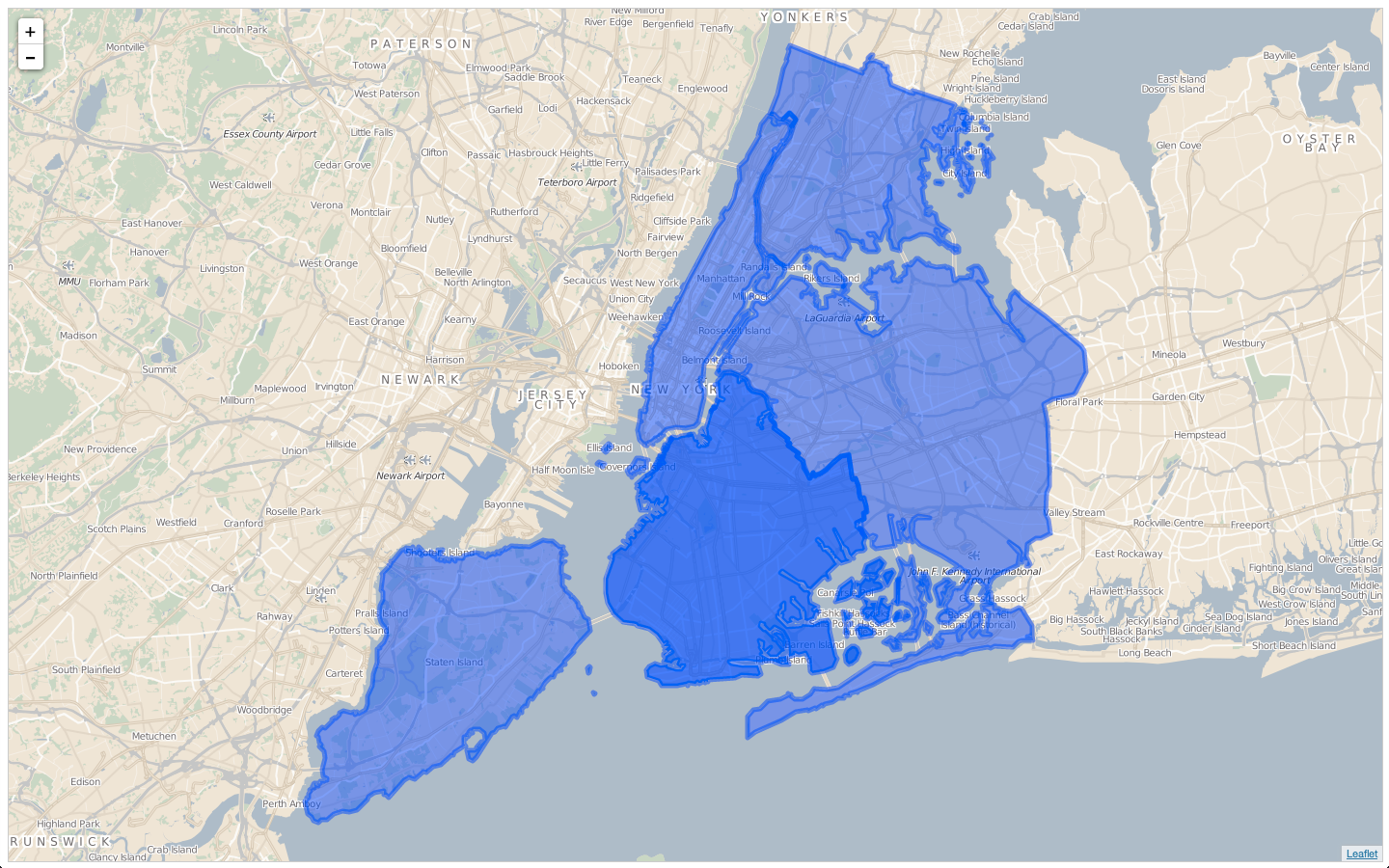```boros.to_json()
```

## Geocoding

```>>> g = geocode(['1900 University Ave, Austin, TX',
'515 Congress Ave, Austin, TX',
'Texas State Capitol, Austin'])
>>> print(g)
0  1900 University Avenue, The University of Texa...
1         515 Congress Avenue, Austin, TX 78701, USA
2  Texas Capitol, 1100 Congress Avenue, Austin, T...

geometry
0         POINT (-97.74034189999999 30.2824039)
1  POINT (-97.74252039999999 30.26759109999999)
2         POINT (-97.74035049999999 30.2746652)
```

## GeoPandas example

`examples/GeoDataFrame example.ipynb`

## GeoPandas exercise

`exercises/Idaho lakes.ipynb`

## Spatial joins

```joined = sjoin(points, boros, how='inner')
```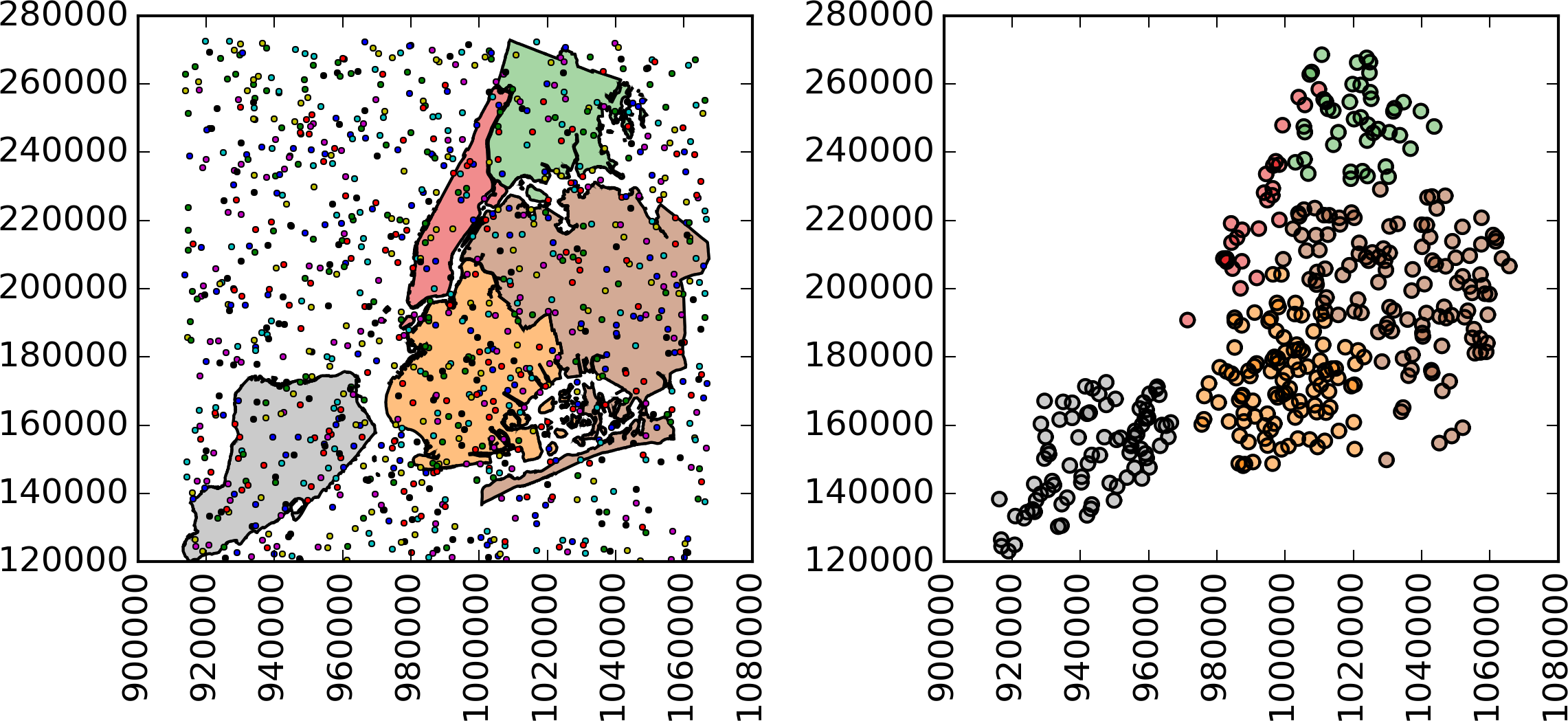`examples/sjoin.py`

contact info: